Explain the usage of analytical functions in hive?
BIG DATA RECIPES DATA CLEANING PYTHON DATA MUNGING MACHINE LEARNING RECIPES PANDAS CHEATSHEET     ALL TAGS

# Explain the usage of analytical functions in hive?

This recipe explains what the usage of analytical functions in hive

0

## Recipe Objective

In big data scenarios , when data volume is huge, we may need to find a subset of data to speed up data analysis. Here comes a technique to select and analyze a subset of data in order to identify patterns and trends in the data known as sampling.

## Step 1 : Prepare the dataset

Here we are using the employee related comma separated values (csv) dataset for the create hive table in local.

Data of Output looks as follows: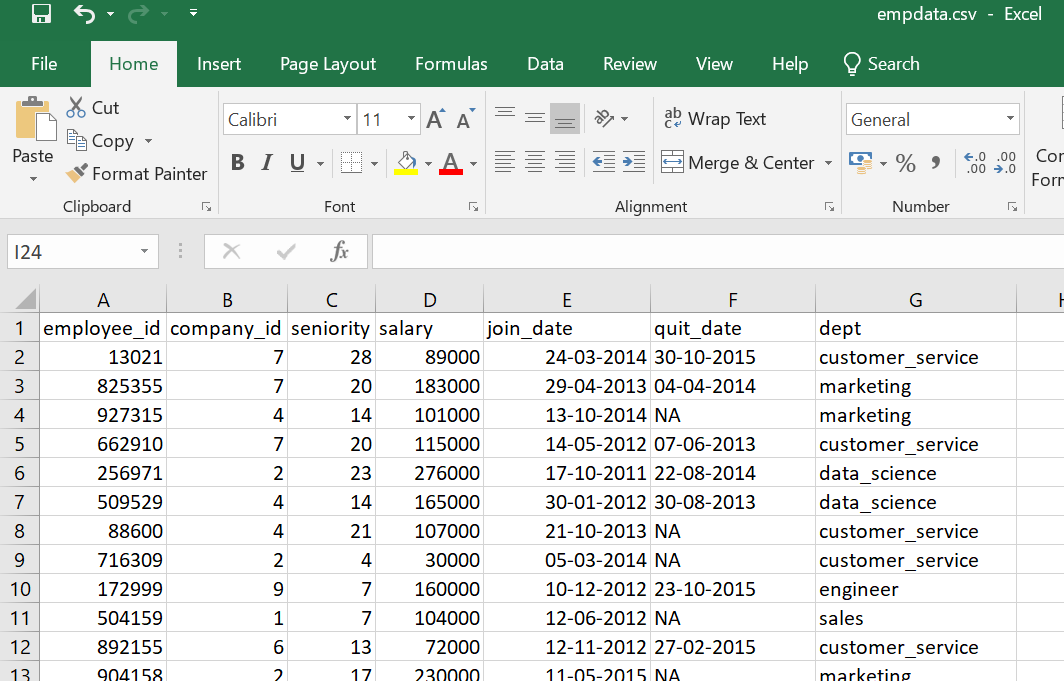Before create a table open the hive shell and we need to create a database as follows :Open the hive shell as below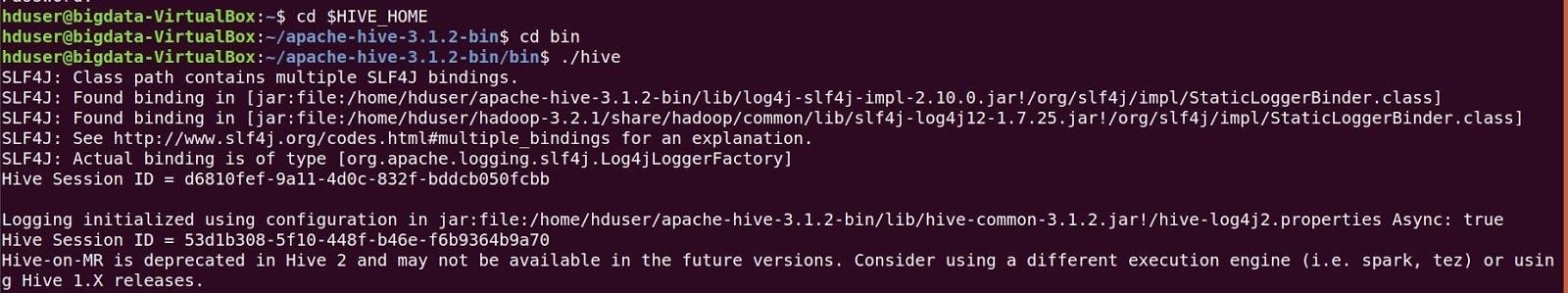To create database using below queries :

``` Create database dezyre_db; use dezyre_db; ```

As follows below: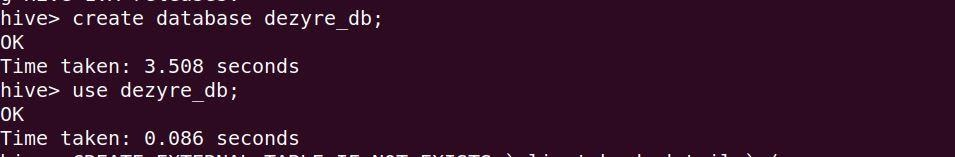## Step 2 : Create a Hive Table and Load the Data into the Table and verify the Data

Here we are going create a hive table for loading the data from this table to created bucketed tables, Use below to create a hive table:

``` CREATE TABLE employee ( employee_id int, company_id int, seniority int, salary int, join_date string, quit_date string, dept string ) ROW FORMAT DELIMITED fields terminated by ',' TBLPROPERTIES ("skip.header.line.count"="1"); ```

the above query runs as follows :Loading the data into hive table and verifying the data

``` load data local inpath '/home/bigdata/Downloads/empdata.csv' into table employee; ```

Verifying the data by running the select query as follows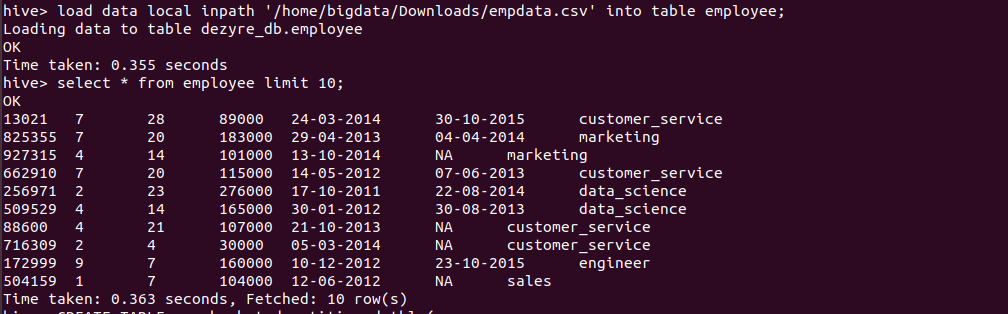## Step 3 : Explanation of Analytic functions in hive

Analytic functions are a special group of functions that scan the multiple input rows to compute each output value. Analytic functions are usually used with OVER, PARTITION BY, ORDER BY, and the windowing specification.The analytic functions offer greater flexibility and functionalities than the regular GROUP BY clause and make special aggregations in Hive easier and powerful.

The Analytic functions are :

• RANK
• DENSE_RANK
• ROW_NUMBER
• CUME_DIST
• PERCENT_RANK
• NTILE

• RANK():
• The rank function ranks items in a group, such as finding the top N rows for specific conditions. This function is used to assign a rank to the rows based on the column values in OVER clause. The row with equal values assigned the same rank with next rank value skipped.

``` hive>SELECT * FROM employee DISTRIBUTE BY RAND() SORT BY RAND() LIMIT 10; ```

Output of the above query :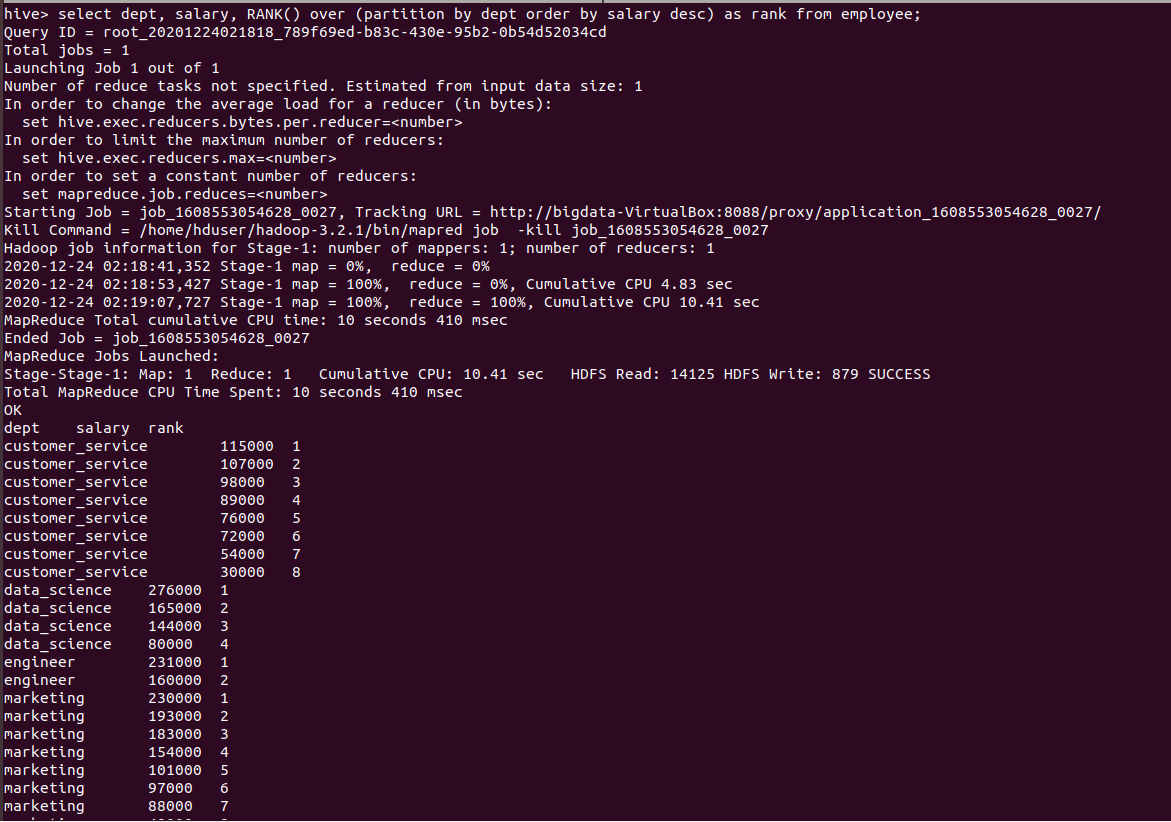• DENSE_RANK():
• It is similar to RANK, but leaves no gaps in the ranking sequence when there are ties. the rank assigns in the sequential order so that no rank values are skipped. DENSE_RANK() function returns consecutive rank values. Rows in each partition receive the same ranks if they have the same values.

``` hive> select dept, salary, DENSE_RANK() over (partition by dept order by salary desc) as dens_rank from employee; ```

the above query runs as follows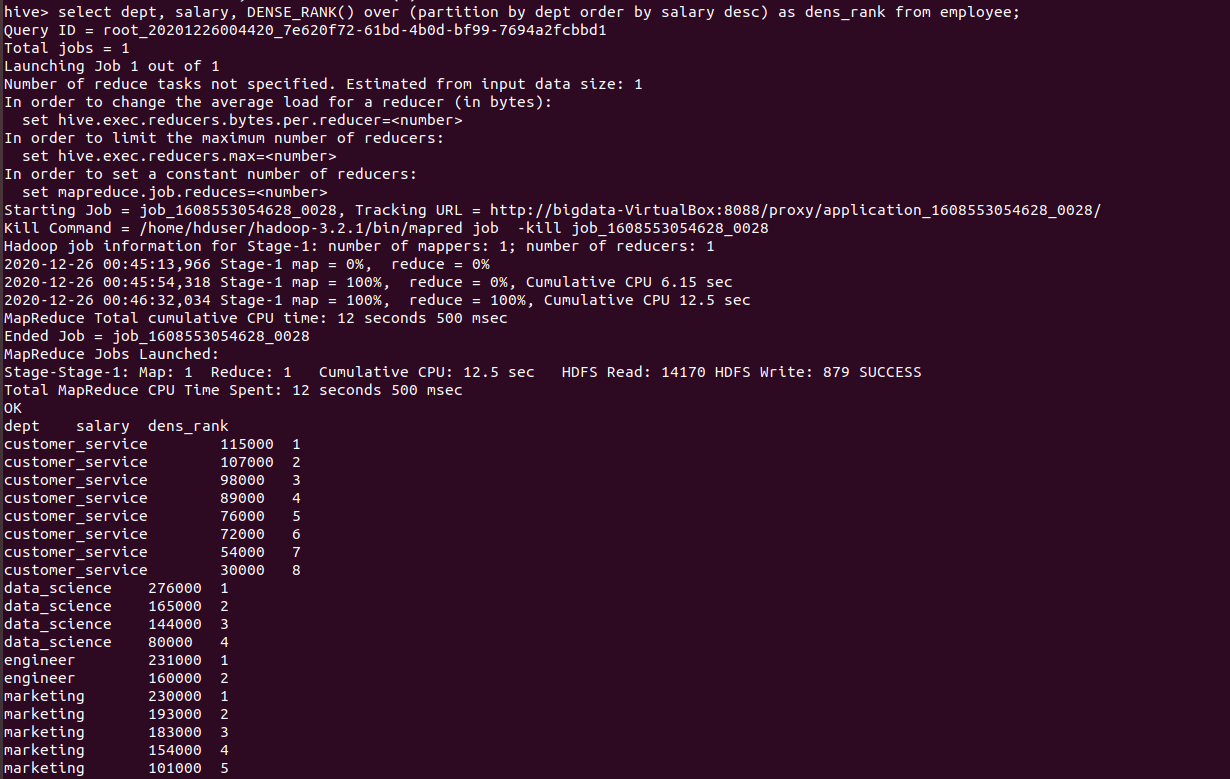• ROW_NUMBER():
• This function is used to assign a unique sequence number starting from 1 to each row according to the partition and order specification.

``` hive> select dept, salary, ROW_NUMBER() over (partition by dept order by salary desc) as row_no from employee; ```

Output of the above query as follows: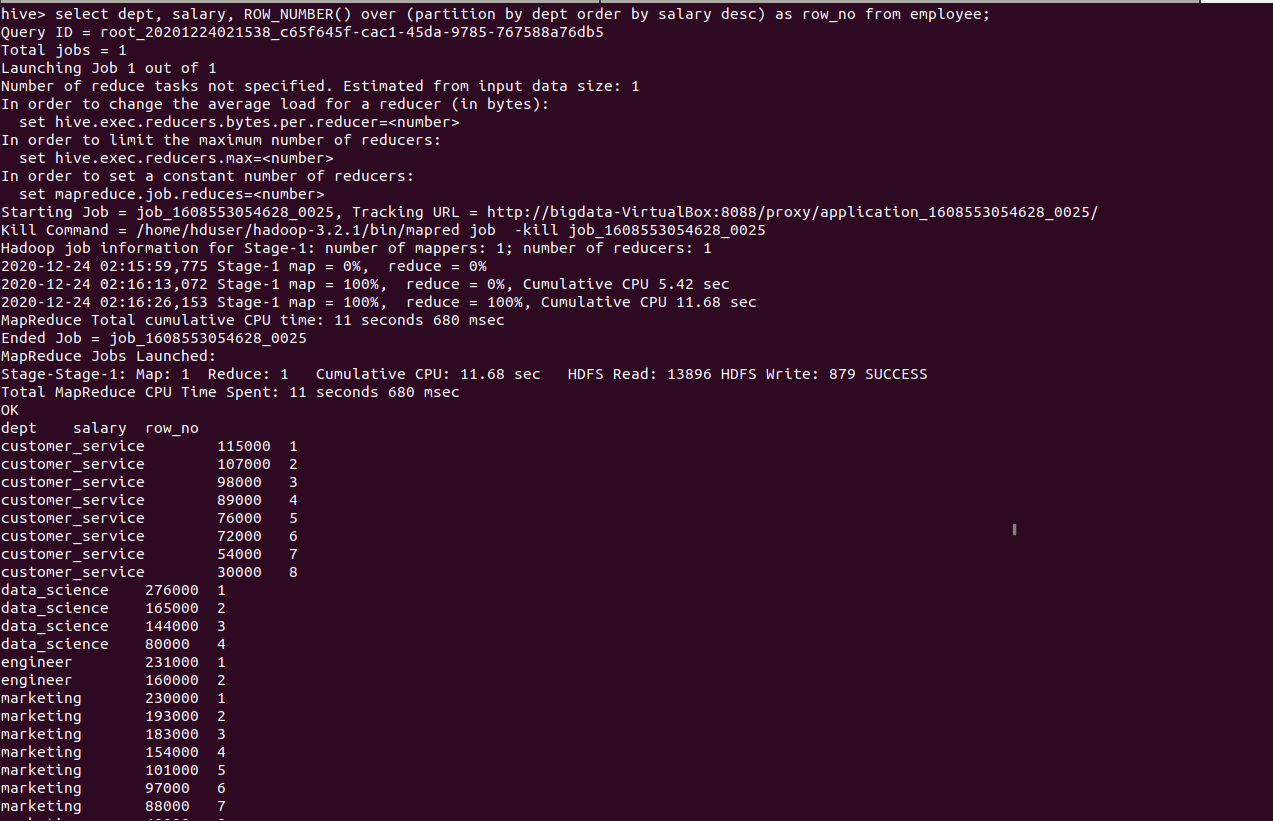• CUME_DIST():
• It computes the number of rows whose value is smaller or equal to the value of the total number of rows divided by the current row.it gives the values in the float data type.

``` hive> SELECT dept, salary, CUME_DIST() OVER (ORDER BY salary) AS cume_dist FROM employee; ```

Output of the above query :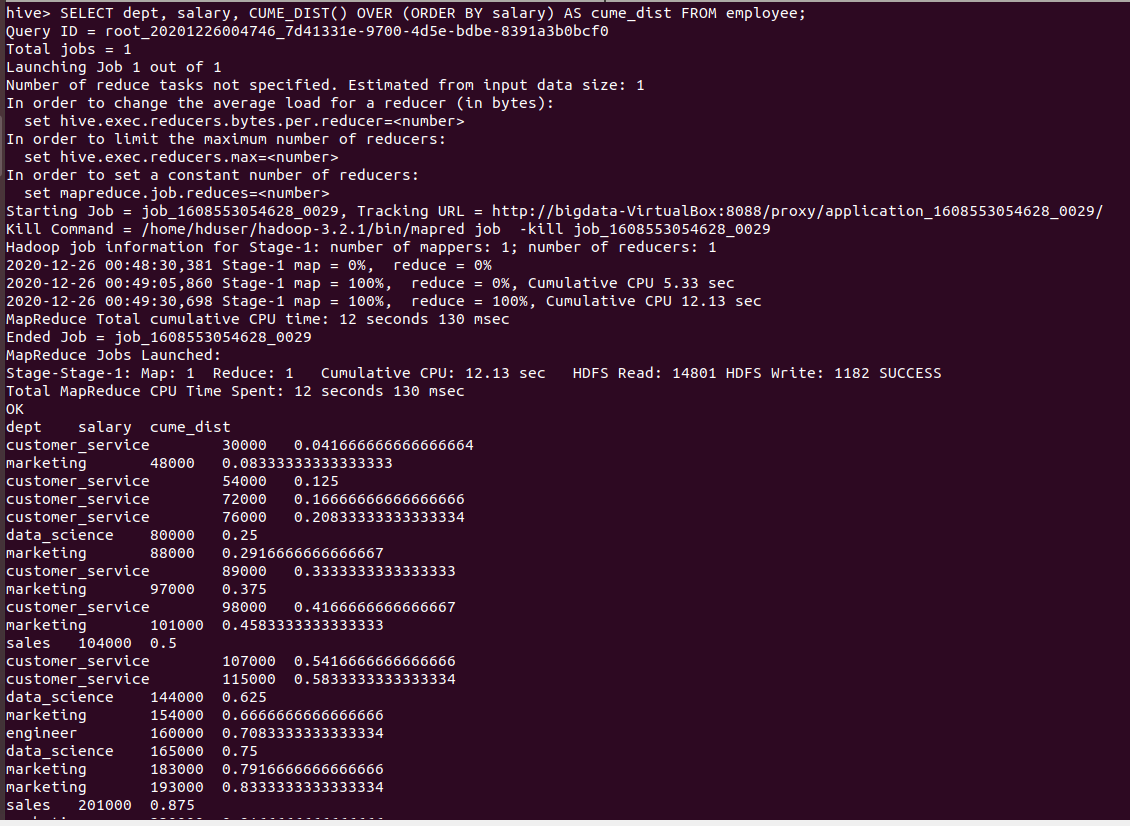• PERCENT_RANK():
• It is similar to CUME_DIST, but it uses rank values rather than row counts in its numerator as total number of rows - 1 divided by current rank - 1. Therefore, it returns the percent rank of a value relative to a group of values.

``` hive>SELECT dept, salary, RANK() OVER (PARTITION BY dept ORDER BY salary DESC) AS rank, PERCENT_RANK() OVER (PARTITION BY dept ORDER BY salary DESC) AS percen_rank FROM employee; ```

Output of the above query :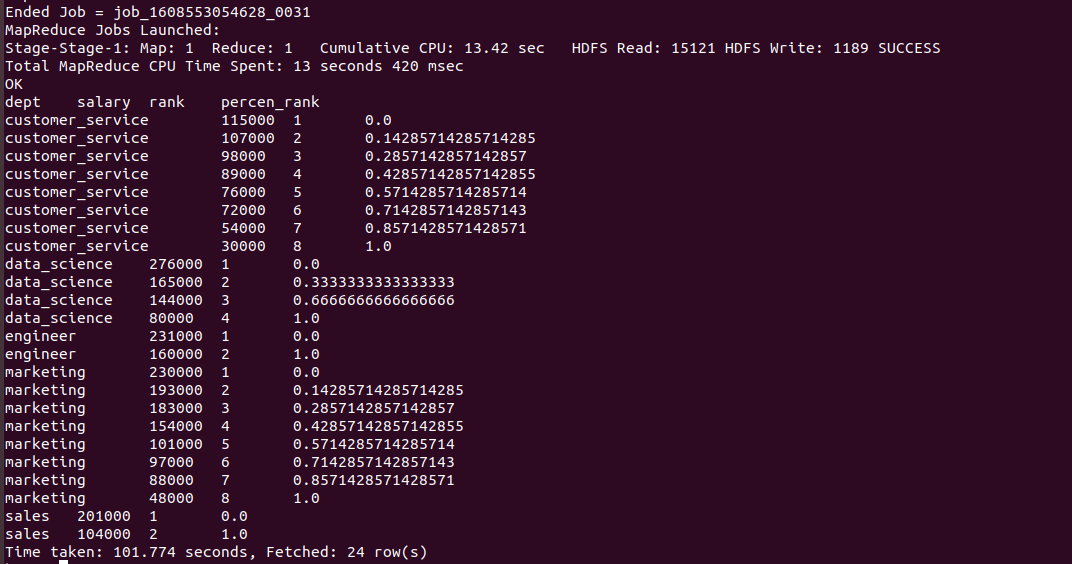• NTILE():
• It divides an ordered dataset into a number of buckets and assigns an appropriate bucket number to each row. It can be used to divide rows into equal sets and assign a number to each row.

``` hive > SELECT dept, salary, NTILE(4) OVER (PARTITION BY dept ORDER BY salary DESC) AS ntile FROM employee; ```

Output of the above query :Performing as query All the analytical funcitons together as follows :

``` hive >SELECT dept AS depart, salary AS sal, ROW_NUMBER() OVER (PARTITION BY dept ORDER BY salary DESC) AS row_num, RANK() OVER (PARTITION BY dept ORDER BY salary DESC) AS rank, DENSE_RANK() OVER(PARTITION BY dept ORDER BY salary DESC) AS dense_rnk, PERCENT_RANK() OVER (PARTITION BY dept ORDER BY salary DESC) AS percen_rank, NTILE(4) OVER (PARTITION BY dept ORDER BY salary DESC) AS ntile FROM employee ; ```

Output of the above query :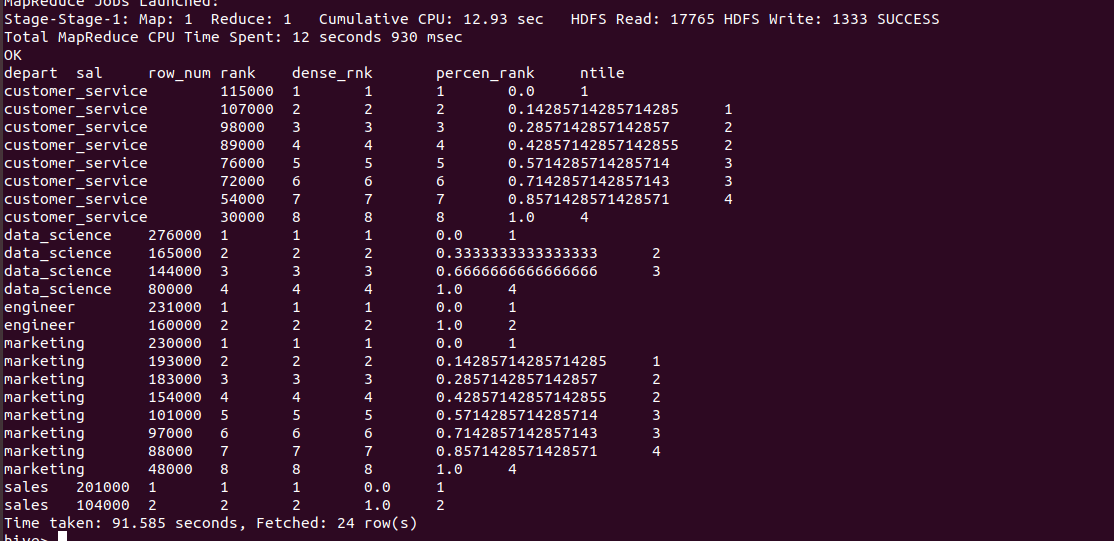#### Relevant Projects

##### Airline Dataset Analysis using Hadoop, Hive, Pig and Impala
Hadoop Project- Perform basic big data analysis on airline dataset using big data tools -Pig, Hive and Impala.

##### Online Hadoop Projects -Solving small file problem in Hadoop
In this hadoop project, we are going to be continuing the series on data engineering by discussing and implementing various ways to solve the hadoop small file problem.

##### Data processing with Spark SQL
In this Apache Spark SQL project, we will go through provisioning data for retrieval using Spark SQL.

##### Hadoop Project-Analysis of Yelp Dataset using Hadoop Hive
The goal of this hadoop project is to apply some data engineering principles to Yelp Dataset in the areas of processing, storage, and retrieval.

##### Real-Time Log Processing using Spark Streaming Architecture
In this Spark project, we are going to bring processing to the speed layer of the lambda architecture which opens up capabilities to monitor application real time performance, measure real time comfort with applications and real time alert in case of security

##### Real-Time Log Processing in Kafka for Streaming Architecture
The goal of this apache kafka project is to process log entries from applications in real-time using Kafka for the streaming architecture in a microservice sense.

##### Tough engineering choices with large datasets in Hive Part - 2
This is in continuation of the previous Hive project "Tough engineering choices with large datasets in Hive Part - 1", where we will work on processing big data sets using Hive.

##### Design a Hadoop Architecture
Learn to design Hadoop Architecture and understand how to store data using data acquisition tools in Hadoop.

##### Spark Project-Analysis and Visualization on Yelp Dataset
The goal of this Spark project is to analyze business reviews from Yelp dataset and ingest the final output of data processing in Elastic Search.Also, use the visualisation tool in the ELK stack to visualize various kinds of ad-hoc reports from the data.

##### Analysing Big Data with Twitter Sentiments using Spark Streaming
In this big data spark project, we will do Twitter sentiment analysis using spark streaming on the incoming streaming data.